Our Discord hit 10K members! 🎉 Meet students and ask top educators your questions.Join Here!

## Educators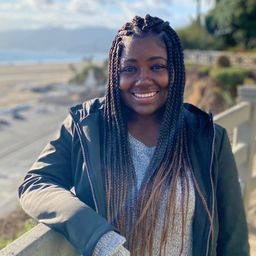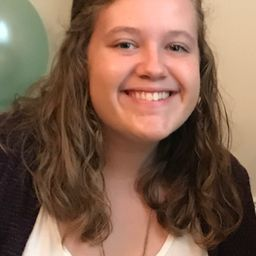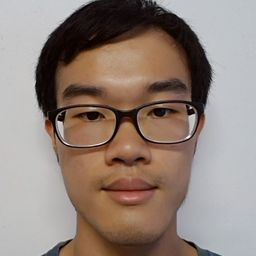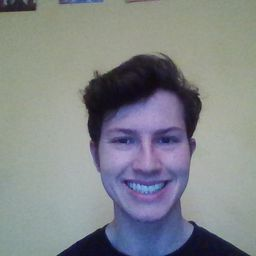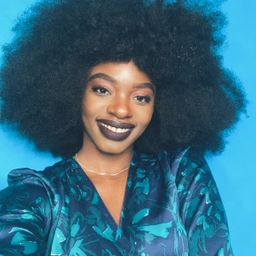+ 3 more educators

### Problem 1

Draw graph models, stating the type of graph (from Table 1 ) used, to represent airline routes where every day there are four flights from Boston to Newark, two flights from Newark to Boston, three flights from Newark to Miami, two flights from Miami to Newark, one flight from Newark to Detroit, two flights from Detroit to Newark, three flights from Newark to Washington, two
flights from Washington to Newark, and one flight from Washington to Miami, with
a) an edge between vertices representing cities that have a flight between them (in either direction).
b) an edge between vertices representing cities for each flight that operates between them (in either direction).
c) an edge between vertices representing cities for each flight that operates between them (in either direction), plus a loop for a special sightseeing trip that takes off and lands in Miami.
d) an edge from a vertex representing a city where a flight starts to the vertex representing the city where it ends.
e) an edge for each flight from a vertex representing a city where the flight begins to the vertex representing the city where the flight ends.Jenna D.
Numerade Educator

### Problem 2

What kind of graph (from Table 1) can be used to model a highway system between major cities where
a) there is an edge between the vertices representing cities if there is an interstate highway between them?
b) there is an edge between the vertices representing cities for each interstate highway between them?
c) there is an edge between the vertices representing cities for each interstate highway between them, and there is a loop at the vertex representing a city if there is an interstate highway that circles this city?Prathan J.
Numerade Educator

### Problem 3

For Exercises $3-9$ , determine whether the graph shown has directed or undirected edges, whether it has multiple edges, and whether it has one or more loops. Use your answers to determine the type of graph in Table 1 this graph is.
(GRAPH NOT COPY)Chris T.
Numerade Educator

### Problem 4

For Exercises $3-9$ , determine whether the graph shown has directed or undirected edges, whether it has multiple edges, and whether it has one or more loops. Use your answers to determine the type of graph in Table 1 this graph is.
(GRAPH NOT COPY)Prathan J.
Numerade Educator

### Problem 5

For Exercises $3-9$ , determine whether the graph shown has directed or undirected edges, whether it has multiple edges, and whether it has one or more loops. Use your answers to determine the type of graph in Table 1 this graph is.
(GRAPH NOT COPY)Chris T.
Numerade Educator

### Problem 6

For Exercises $3-9$ , determine whether the graph shown has directed or undirected edges, whether it has multiple edges, and whether it has one or more loops. Use your answers to determine the type of graph in Table 1 this graph is.
(GRAPH NOT COPY)Prathan J.
Numerade Educator

### Problem 7

For Exercises $3-9$ , determine whether the graph shown has directed or undirected edges, whether it has multiple edges, and whether it has one or more loops. Use your answers to determine the type of graph in Table 1 this graph is.
(GRAPH NOT COPY)Chris T.
Numerade Educator

### Problem 8

For Exercises $3-9$ , determine whether the graph shown has directed or undirected edges, whether it has multiple edges, and whether it has one or more loops. Use your answers to determine the type of graph in Table 1 this graph is.
(GRAPH NOT COPY)Prathan J.
Numerade Educator

### Problem 9

For Exercises $3-9$ , determine whether the graph shown has directed or undirected edges, whether it has multiple edges, and whether it has one or more loops. Use your answers to determine the type of graph in Table 1 this graph is.
(GRAPH NOT COPY)Noah M.
Numerade Educator

### Problem 10

For each undirected graph in Exercises $3-9$ that is not simple, find a set of edges to remove to make it simple.Prathan J.
Numerade Educator

### Problem 11

Let $G$ be a simple graph. Show that the relation $R$ on the set of vertices of $G$ such that $u R v$ if and only if there is an edge associated to $\{u, v\}$ is a symmetric, irreflexive relation on $G .$Chris T.
Numerade Educator

### Problem 12

Let $G$ be an undirected graph with a loop at every vertex. Show that the relation $R$ on the set of vertices of $G$ such that $u R v$ if and only if there is an edge associated to $\{u, v\}$ is a symmetric, reflexive relation on $G .$Prathan J.
Numerade Educator

### Problem 13

The intersection graph of a collection of sets $A_{1}$ , $A_{2}, \ldots, A_{n}$ is the graph that has a vertex for each of these sets and has an edge connecting the vertices representing two sets if these sets have a nonempty intersection. Construct the intersection graph of these collections of sets.
a) $A_{1}=\{0,2,4,6,8\}, A_{2}=\{0,1,2,3,4\}$
$A_{3}=\{1,3,5,7,9\}, A_{4}=\{5,6,7,8,9\}$
$A_{5}=\{0,1,8,9\}$
b) $A_{1}=\{\ldots,-4,-3,-2,-1,0\}$
$A_{2}=\{\ldots,-2,-1,0,1,2, \ldots\}$
$A_{3}=\{\ldots,-6,-4,-2,0,2,4,6, \ldots\}$
$A_{4}=\{\ldots,-5,-3,-1,1,3,5, \ldots\}$
$A_{5}=\{\ldots,-6,-3,0,3,6, \ldots\}$
c) $A_{1}=\{x | x < 0\}$
$A_{2}=\{x |-1 < x < 0\}$
$A_{3}=\{x | 0 < x < 1\}$
$A_{4}=\{x |-1 < x < 1\}$
$A_{5}=\{x | x > -1\}$
$A_{6}=\mathbf{R}$Chris T.
Numerade Educator

### Problem 14

Use the niche overlap graph in Figure 11 to determine the species that compete with hawks.Prathan J.
Numerade Educator

### Problem 15

Construct a niche overlap graph for six species of birds, where the hermit thrush competes with the robin and with the blue jay, the robin also competes with the mockingbird, the mockingbird also competes with the blue jay, and the nuthatch competes with the hairy wood- pecker.Chris T.
Numerade Educator

### Problem 16

Draw the acquaintanceship graph that represents that Tom and Patricia, Tom and Hope, Tom and Sandy, Tom and Amy, Tom and Marika, Jeff and Patricia, Jeff and Mary, Patricia and Hope, Amy and Hope, and Amy and Marika know each other, but none of the other pairs of people listed know each other.Prathan J.
Numerade Educator

### Problem 17

We can use a graph to represent whether two people were alive at the same time. Draw such a graph to represent whether each pair of the mathematicians and computer scientists with biographies in the first five chapters of this book who died before 1900 were contemporaneous. (Assume two people lived at the same time if they were alive during the same year.)

JS
Jamie S.
Numerade Educator

### Problem 18

Who can influence Fred and whom can Fred influence in the influence graph in Example 2$?$Prathan J.
Numerade Educator

### Problem 19

Construct an influence graph for the board members of a company if the President can influence the Director of Research and Development, the Director of Marketing, and the Director of Operations; the Director of Research and Development can influence the Director of Operations; the Director of Marketing can influence the Director of Operations; and no one can influence, or be influenced by, the Chief Financial Officer.Chris T.
Numerade Educator

### Problem 20

The word apple can refer to a plant, a food, or a computer company. Construct a word graph for these nouns: apple, strawberry, lenovo, cheese, chocolate, ibm, oak, microsoft, hedge, grass, cake, quiche, hp, cider, donut, azalea, pine, dell, fir, raspberry. Connect two vertices by an undirected edge if the nouns they represent have similar meaning.Chris T.
Numerade Educator

### Problem 21

The word rock can refer to a type of music or to something from a mountain. Construct a word graph for
these nouns: rock, boulder, jazz, limestone, gravel, folk, bachata, pumice, granite, tango, klezmer, slate, shale, classical, pebbles, sand, rap, marble. Connect two vertices by an undirected edge if the nouns they represent have similar meaning.Noah M.
Numerade Educator

### Problem 22

Which other teams did Team 4 beat and which teams beat Team 4 in the round-robin tournament represented by the graph in Figure 14$?$Prathan J.
Numerade Educator

### Problem 23

In a round-robin tournament the Tigers beat the Blue Jays, the Tigers beat the Cardinals, the Tigers beat the Orioles, the Blue Jays beat the Cardinals, the Blue Jays beat the Orioles, and the Cardinals beat the Orioles. Model this outcome with a directed graph.Chris T.
Numerade Educator

### Problem 24

Construct the call graph for a set of seven telephone numbers $555-0011,555-1221,555-1333,555-8888$
$555-2222,555-0091,$ and $555-1200$ if there were three calls from $555-0011$ to $555-8888$ and two calls from $555-8888$ to $555-0011$ , two calls from $555-2222$ to $555-0091,$ two calls from $555-1221$ to each of the other numbers, and one call from $555-1333$ to each of $555-0011,555-1221,$ and $555-1200$Prathan J.
Numerade Educator

### Problem 25

Explain how the two telephone call graphs for calls made during the month of January and calls made during the month of February can be used to determine the new telephone numbers of people who have changed their telephone numbers.Chris T.
Numerade Educator

### Problem 26

a) Explain how graphs can be used to model electronic mail messages in a network. Should the edges be directed or undirected? Should multiple edges be allowed? Should loops be allowed?
b) Describe a graph that models the electronic mail sent in a network in a particular week.Chris T.
Numerade Educator

### Problem 27

How can a graph that models e-mail messages sent in a network be used to find people who have recently
changed their primary e-mail address?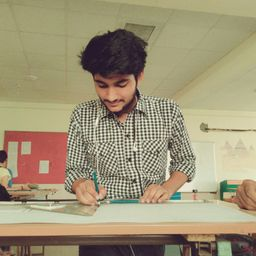Prashant B.
Numerade Educator

### Problem 28

How can a graph that models e-mail messages sent in a network be used to find electronic mail mailing lists used to send the same message to many different e-mail addresses?

Check back soon!

### Problem 29

Describe a graph model that represents whether each person at a party knows the name of each other person at the party. Should the edges be directed or undirected? Should multiple edges be allowed? Should loops be allowed?Chris T.
Numerade Educator

### Problem 30

Describe a graph model that represents a subway system in a large city. Should edges be directed or undirected? Should multiple edges be allowed? Should loops be allowed?Prathan J.
Numerade Educator

### Problem 31

For each course at a university, there may be one or more other courses that are its prerequisites. How can a graph be used to model these courses and which courses are prerequisites for which courses? Should edges be directed or undirected? Looking at the graph model, how can we find courses that do not have any prerequisites and how can we find courses that are not the prerequisite for any other courses?

Check back soon!

### Problem 32

Describe a graph model that represents the positive recommendations of movie critics, using vertices to represent both these critics and all movies that are currently being shown.Prathan J.
Numerade Educator

### Problem 33

Describe a graph model that represents traditional marriages between men and women. Does this graph have any special properties?Chris T.
Numerade Educator

### Problem 34

Which statements must be executed before $S_{6}$ is executed in the program in Example 8$?$ (Use the precedence graph in Figure $10 . )$Chris T.
Numerade Educator

### Problem 35

Construct a precedence graph for the following program:
$$\begin{array}{l}{S_{1} : x :=0} \\ {S_{2} : x :=x+1} \\ {S_{3} : y :=2} \\ {S_{4} : z :=y} \\ {S_{5} : x :=x+2} \\ {S_{6} : x :=x+z} \\ {S_{6} : y :=x+z} \\ {S_{7} : z :=4}\end{array}$$Chris T.
Numerade Educator

### Problem 36

Describe a discrete structure based on a graph that can be used to model airline routes and their flight times. $[\text { Hint: Add structure to a directed graph. }]$Prathan J.
Numerade Educator

### Problem 37

Describe a discrete structure based on a graph that can be used to model relationships between pairs of individuals in a group, where each individual may either like, dislike, or be neutral about another individual, and the reverse relationship may be different. [Hint: Add structure to a directed graph. Treat separately the edges in opposite directions between vertices representing two individuals. $]$Chris T.
Numerade Educator

### Problem 38

Describe a graph model that can be used to represent all forms of electronic communication between two people in a single graph. What kind of graph is needed?Prathan J.
Numerade Educator